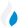•Trade Pi
•Trade
•Exchange
•Trade Pi
•Trade
•Exchange
\$1.12T
Total marketcap
\$73.2B
Total volume
39.45%
BTC dominance
•Trade Pi
•Trade
•Exchange

# alice to TRY Exchange Rate - 1 My Neighbor Alice in TRY

38.849833885358
• BTC 0.00009
• ETH 0.0012
Vol [24h]
\$824,734.38

## alice to TRY converter

Exchange Pair Price 24h volume
Binance ALICE/TRY \$2.07 \$574.05K
Paribu ALICE/TRY \$2.04 \$242.96K
Bitlo ALICE/TRY \$2.02 \$10.41K
Nominex ALICE/TRY \$2.06 \$594.59

## ALICE/TRY Exchange Rate Overview

Name Ticker Price % 24h 24h high 24h low 24h volume
My Neighbor Alice alice \$2.02 -5.8179% \$2.17 \$1.99 \$74.78M

Selling 1 My Neighbor Alice alice you get 38.849833885358 TRY.

My Neighbor Alice Mar 15, 2021 had the highest price, at that time trading at its all-time high of \$40.93.

696 days have passed since then, and now the price is 4.96% of the maximum.

Based on the table data, the ALICE vs TRY exchange volume is \$824,734.38.

Using the calculator/converter on this page, you can make the necessary calculations with a pair of My Neighbor Alice to TRY.

## Q&A

### What is the current ALICE to TRY exchange rate?

Right now, the ALICE/TRY exchange rate is 38.849833885358.

### What has been the My Neighbor Alice to TRY trading volume in the last 24 hours?

Relying on the table data, the My Neighbor Alice to TRY exchange volume is \$824,734.38.

### How can I calculate the amount of TRY? / How do I convert my My Neighbor Alice to TRY?

You can calculate/convert TRY from ALICE to TRY converter. Also, you can select other currencies from the drop-down list.

## ALICE to TRY Сonversion Table

ALICE TRY
0.2 ALICE = 7.7 TRY
0.4 ALICE = 15.5 TRY
0.9 ALICE = 34.9 TRY
1 ALICE = 38.8 TRY
2 ALICE = 77.6 TRY
5 ALICE = 194.2 TRY
7 ALICE = 271.9 TRY
30 ALICE = 1,165.4 TRY
50 ALICE = 1,942.4 TRY
500 ALICE = 19,424.9 TRY
5000 ALICE = 194,249.1 TRY
50000 ALICE = 1,942,491.6 TRY
500000 ALICE = 19,424,916.9 TRY